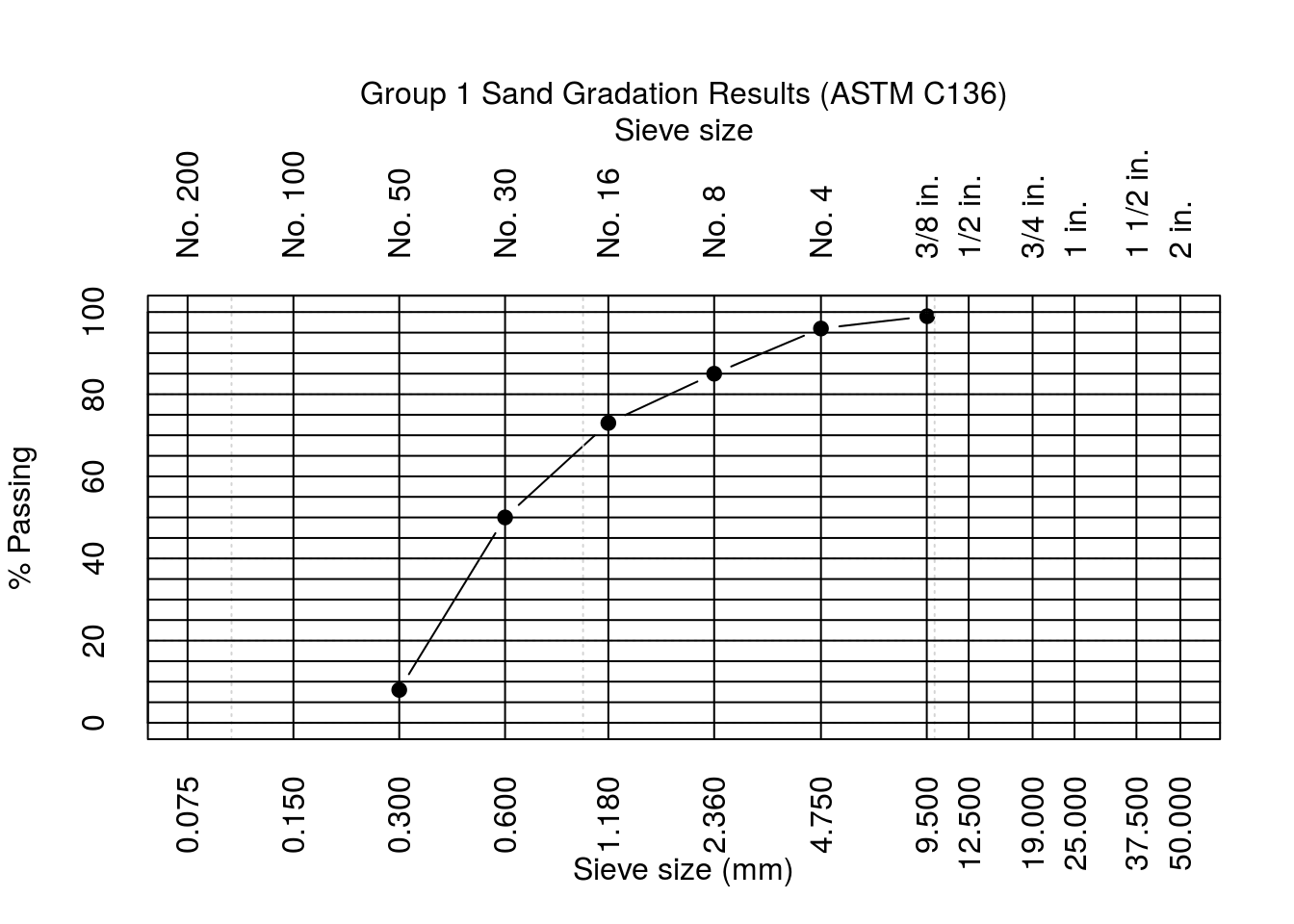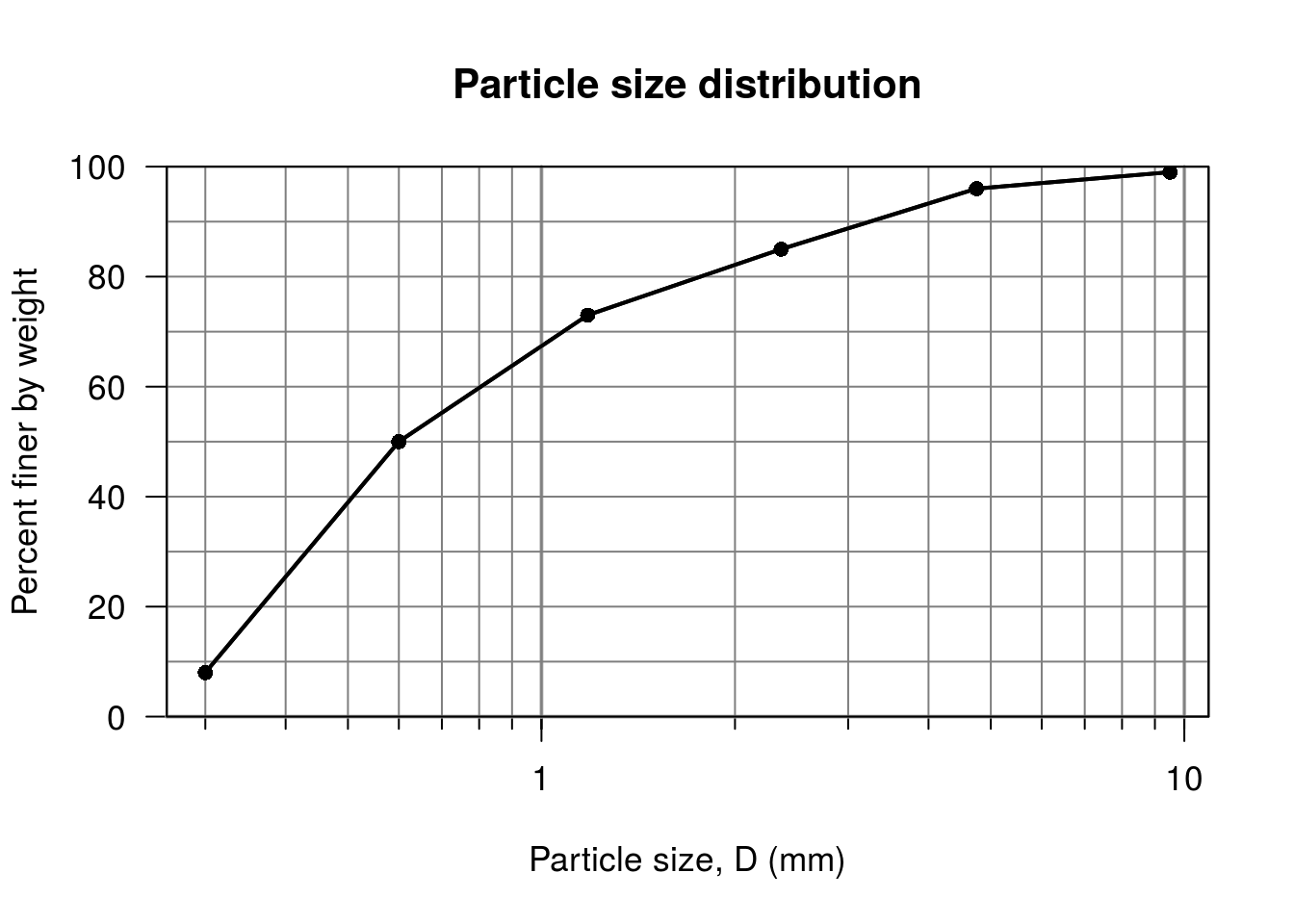Note: If you wish to replicate the R code below, then you will need to copy and paste the following commands in R first (to make sure you have all the packages and their dependencies):

``````install.packages("install.load")

# install and/or load the packages and their dependencies

install.packages("import")
# install the import package

import::from(pracma, semilogx, std)
# import semilogx and std from the pracma package``````

This document was created with rmarkdown 1.2 using the following:

• R 3.3.2 (2016-10-31)
• htmlTable 1.7
• data.table 1.9.8
• NISTunits 1.0.1
• geotech 1.0
• ggplot2 2.2.0
• import 1.1.0
• pracma 1.9.5

# Sand Gradation Analysis Examples from Tennessee State University (TSU) Structural Testing Laboratory undergraduate-level course, Fall 2014 and Fall 2016

``````install.load::load_package("geotech", "ggplot2", "data.table", "htmlTable",
"NISTunits")
# package (it is assumed that you have already installed these packages)

import::from(pracma, semilogx, std)
# import semilogx and std from the pracma package

# Example 1 from Fall 2014 Group 1

sand_grad_1a <- data.table(Sieve = c("3/8”", "4", "8", "16", "30", "50", "Pan"),
Size = c(NISTinchTOcm(3/8) * 10, 4.75, 2.36, 1.18, 0.6, 0.3, NA), Mass_Sieve = c(0.516,
0.734, 0.46, 0.408, 0.39, 0.344, 0.362), Mass_SievePlus = c(0.516, 0.766,
0.566, 0.532, 0.618, 0.76, 0.45))
set(sand_grad_1a, i = 7L, j = c(5L:8L), value = NA)
setnames(sand_grad_1a, c("Sieve #", "Size (mm)", "Mass Sieve (kg)", "Mass Retained + Sieve (kg)",
"Mass Retained (kg)", "Cumulative Mass Retained (kg)", "Cumulative % Retained",
"% Passing"))

htmlTable(sand_grad_1a, rnames = FALSE, align = "c", align.header = "l", caption = "Fall 2014 Group 1 Sieve Analysis Table",
 Sieve # Size (mm) Mass Sieve (kg) Mass Retained + Sieve (kg) Mass Retained (kg) Cumulative Mass Retained (kg) Cumulative % Retained % Passing Fall 2014 Group 1 Sieve Analysis Table 3/8” 9.525 0.516 0.516 0 0 0 100 4 4.75 0.734 0.766 0.032 0.032 3 97 8 2.36 0.46 0.566 0.106 0.138 14 86 16 1.18 0.408 0.532 0.124 0.262 26 74 30 0.6 0.39 0.618 0.228 0.49 49 51 50 0.3 0.344 0.76 0.416 0.906 91 9 Pan 0.362 0.45
``````# calculate the Fineness Modulus
FM_1a <- sum(sand_grad_1a[, 7], na.rm = TRUE)/100

FM_1a``````
``##  1.83``
``````op <- par(mar = c(5, 4, 8, 2))  # set margins
ylab = "% Passing", type = "b", axes = FALSE, pch = 19, xlim = c(0.075,
50), ylim = c(0, 100))
x1 <- c(0.075, 0.15, 0.3, 0.6, 1.18, 2.36, 4.75, 9.5, 12.5, 19, 25, 37.5, 50)
x2 <- c("No. 200", "No. 100", "No. 50", "No. 30", "No. 16", "No. 8", "No. 4",
"3/8 in.", "1/2 in.", "3/4 in.", "1 in.", "1 1/2 in.", "2 in.")
y <- seq(0, 100, 10)
axis(1, at = x1, labels = NULL, tck = 0, las = 2)
axis(3, at = x1, labels = x2, tck = 0, las = 2)
abline(h = seq(0, 100, 5), v = x1)
axis(2, at = y, labels = y, tck = 0)
box()  # Source 3
mtext("Sieve size", side = 3, line = 4, cex.lab = 0.8, las = 0)
mtext("Group 1 Sand Gradation Results (ASTM C136)", side = 3, line = 5, cex.lab = 0.8,
las = 0)````````````par(op)  # reset to previous settings

sieve <- c(3/8, 4, 8, 16, 30, 50)

# Modified Example 1 from the grainSize function of the geotech package Plot
# grain-size distribution
1L), 8] - 1L), metric = TRUE)````````````# Group 2

sand_grad_2a <- data.table(Sieve = c("3/8”", "4", "8", "16", "30", "50", "Pan"),
Size = c(NISTinchTOcm(3/8)/100, 4.75, 2.36, 1.18, 0.6, 0.3, NA), Mass_Sieve = c(0.516,
0.508, 0.462, 0.406, 0.38, 0.348, 0.366), Mass_SievePlus = c(0.516,
0.538, 0.558, 0.522, 0.606, 0.77, 0.466))
set(sand_grad_2a, i = 7L, j = c(5L:8L), value = NA)
setnames(sand_grad_2a, c("Sieve #", "Size (mm)", "Mass Sieve (kg)", "Mass Retained + Sieve (kg)",
"Mass Retained (kg)", "Cumulative Mass Retained (kg)", "Cumulative % Retained",
"% Passing"))

htmlTable(sand_grad_2a, rnames = FALSE, align = "c", align.header = "l", caption = "Fall 2014 Group 2 Sieve Analysis Table",
 Sieve # Size (mm) Mass Sieve (kg) Mass Retained + Sieve (kg) Mass Retained (kg) Cumulative Mass Retained (kg) Cumulative % Retained % Passing Fall 2014 Group 2 Sieve Analysis Table 3/8” 0.009525 0.516 0.516 0 0 0 100 4 4.75 0.508 0.538 0.03 0.03 3 97 8 2.36 0.462 0.558 0.096 0.126 13 87 16 1.18 0.406 0.522 0.116 0.242 24 76 30 0.6 0.38 0.606 0.226 0.468 47 53 50 0.3 0.348 0.77 0.422 0.89 90 10 Pan 0.366 0.466
``````# calculate the Fineness Modulus
FM_2a <- sum(sand_grad_2a[, 7], na.rm = TRUE)/100

FM_2a``````
``##  1.77``
``````# mean, standard deviation, and variance of the Fineness Modulus (Group 1
# and 2)
FM_2014 <- c(FM_1a, FM_2a)

mean(FM_2014)``````
``##  1.8``
``std(FM_2014)``
``##  0.04242641``
``var(FM_2014)``
``##  0.0018``
``````op <- par(mar = c(5, 4, 8, 2))  # set margins
semilogx(sand_grad_2a[, 2], sand_grad_2a[, 8], xlab = "Sieve size (mm)", ylab = "% Passing",
type = "b", axes = FALSE, pch = 19, xlim = c(0.075, 50), ylim = c(0, 100))
axis(1, at = x1, labels = NULL, tck = 0, las = 2)
axis(3, at = x1, labels = x2, tck = 0, las = 2)
axis(2, at = y, labels = y, tck = 0)
abline(h = seq(0, 100, 5), v = x1)
box()  # Source 3
mtext("Sieve size", side = 3, line = 4, cex.lab = 0.8, las = 0)
mtext("Group 2 Sand Gradation Results (ASTM C136)", side = 3, line = 5, cex.lab = 0.8,
las = 0)``````